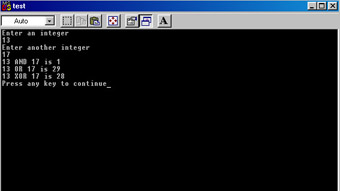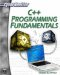# Bitwise Operations

The bitwise operators are a bit more difficult to understand than standard operators (pun intended). The first order of business is to define what a bit is. The computer ultimately stores everything as either a 1 or a 0. A single value of either 1 or 0 is called a bit. Essentially C++ allows you to work directly with the binary equivalents of numbers, to work with bits. This is useful in many computer applications, especially in the telecommunications industry. You will also see these operations in a later chapter when we explore basic encryption algorithms. Explaining bitwise operations necessitates first explaining binary numbers.

The numbers you are familiar with in day to day usage are decimal numbers, base 10 numbers. The base 10 number system is only one possible number system. You can use base 2, base 5, base 16, or any other base you wish. The reason most human societies have always used base 10 number systems is that we have ten fingers. Therefore, our ancestors had an easier time counting in tens, thus number systems started out being based on 10. A computer, however, “speaks” in on or off, base 2 numbers (also called binary numbers). For this reason a basic understanding of binary numbers is essential for programming. In the base 2 number system you can only have 0’s and 1’s. (Notice you don’t actually have any 2’s, just as the base 10 system only has 0’s to 9’s.) What this means is that after you have placed a zero or a one in a particular place, to increase the number you have to move over to a new place. This table (Table 3.4) might help clarify that a bit.

Table 3.4: Binary Numbers

Base 10 Number

Base 2 Equivalent

0

0

1

1

2

10 (Note this is a 1 in the 2’s place and a 0 in the 1’s place, or one 2 and no 1’s.)

3

11 (This is a 1 in the 2’s place and a 1 in the 1’s place, thus equaling one 2 and one 1, which is 3.)

4

100 (This is a 1 in the 4’s place, a 0 in the 2’s place, and a 0 in the 1’s place.)

5

101 (This is a 1 in the 4’s place, a 0 in the 2’s place, and a 1 in the 1’s place.)

6

110 (This is a 1 in the 4’s place, a 1 in the 2’s place, and a 0 in the 1’s place.)

7

111 (This is a 1 in the 4’s place, a 1 in the 2’s place, and a 1 in the 1’s place. And 4 plus 2 plus 1 equals 7.)

8

1000 (This is a 1 in the 8’s place with a 0 in the 4’s, 2’s, and 1’s places.)

Hopefully you are noticing a pattern here. The places in any number system are essentially the number system’s base raised to some power. For example, in the base 10 system you have a 1’s place, 10’s place, 100’s place, 1000’s place, and so on. If you think about this for just a moment you should realize that 1 is simply 100, 10 is 101, 100 is 102, 1000 is 103, and so on. The same is true for the base 2 number system, where you have a 1’s place, 2’s place, 4’s place, 8’s place, 16’s place, and so on. 1 is simply 20, 2 is 21, 4 is 22, 8 is 23, 16 is 24, 32 is 25 and so forth.

In computers everything is stored in groups of eight bits, called bytes. For this reason all binary numbers are actually represented by an eight-digit series of bits. So that one is represented as 00000001, two as 00000010, three as 00000011, and so on.

Now that you have a basic understanding of what binary numbers are, we can explore bitwise operators. Bitwise operators work on integers as if they where binary numbers. Let’s examine the bitwise shift operators first. A bitwise left shift literally shifts all the bits to the left the specified number of places. Consider the following shift operation.

`4 << 1`

This means to take the binary equivalent of 4 (which is 00001000) and shift all the bits over 1 space. This makes the number 00010000. Notice that this shifts it over to an entirely new place, the 8’s place. So operation 4 <<1 actually yields 8. The right shift operator (>>) simply shifts the numbers to the right rather than the left.

The other two bitwise operators (& and |) are of particular use in a variety of applications including such diverse areas as Windows programming, telecommunication, and encryption, as you will see later in this book. The & operators simply asks whether the digits in both numbers are a 1 (and not a 0). For example, 4 & 2 would actually be evaluated in binary as 00001000 & 00000010; to see how this works, compare them vertically.

`00001000 00000010`

You can now see that this would yield 00000000, because in neither case do both numbers have a 1 in the same place. To further clarify this point, let’s compare two other numbers, 5 & 3. In binary this would be the following.

`00001001 00000011`

This would yield 00000001 because only in the final place do the two numbers have a 1 in the same place.

The | (or) operator asks whether either number has a 1 in that particular place. So if you compare 5 | 3 you would have the following.

`00001001 00000011 `

And that would yield 00001011 (which is 7 in decimal numbers).

The ^ operator is called the exclusive or, or XOR operator. It asks if there is a 1 in one of the numbers but not both, at a given place. For example if you consider 7 and 4 and you XOR them you see the following.

`00000111 00000100`

You get 00000011 or 3 because the XOR operation asks if there is a 1 in the place of one number but NOT the other. In other words, the XOR operation is exclusive to one or the other number, but not both, thus the name. The following example will allow you to AND, OR, and XOR integers.

### Example 3.9

Step 1: Enter the following code into your favorite text editor.

`#include <iostream> using namespace std; int main() {     int num1, num2,iand,ior,ixor;  cout << "Enter an integer \n";  cin >> num1;  cout << "Enter another integer \n";  cin >> num2;  iand = num1 & num2;  ior = num1 | num2;  ixor = num1 ^ num2;     cout << num1 << " AND " << num2 << " is " << iand <<   endl;  cout << num1 << " OR " << num2 << " is " << ior <<  endl;  cout << num1 << " XOR " << num2 << " is " << ixor <<  endl;  return 0; }`

Step 2: Compile and run the code. You should see an image much like the one shown in Figure 3.7.Figure 3.7: Bitwise operations.

These binary operators are going to be of particular importance to you later when you encounter encryption, but they are also used in other types of programming. It is also important to understand binary numbers for the simple reason that this is what the computer ultimately translates everything you enter into. The computer only understands binary numbers, so anything you enter into it gets translated into binary.C++ Programming Fundamentals (Cyberrookies)
ISBN: 1584502371
EAN: 2147483647
Year: 2005
Pages: 197
Authors: Chuck Easttom